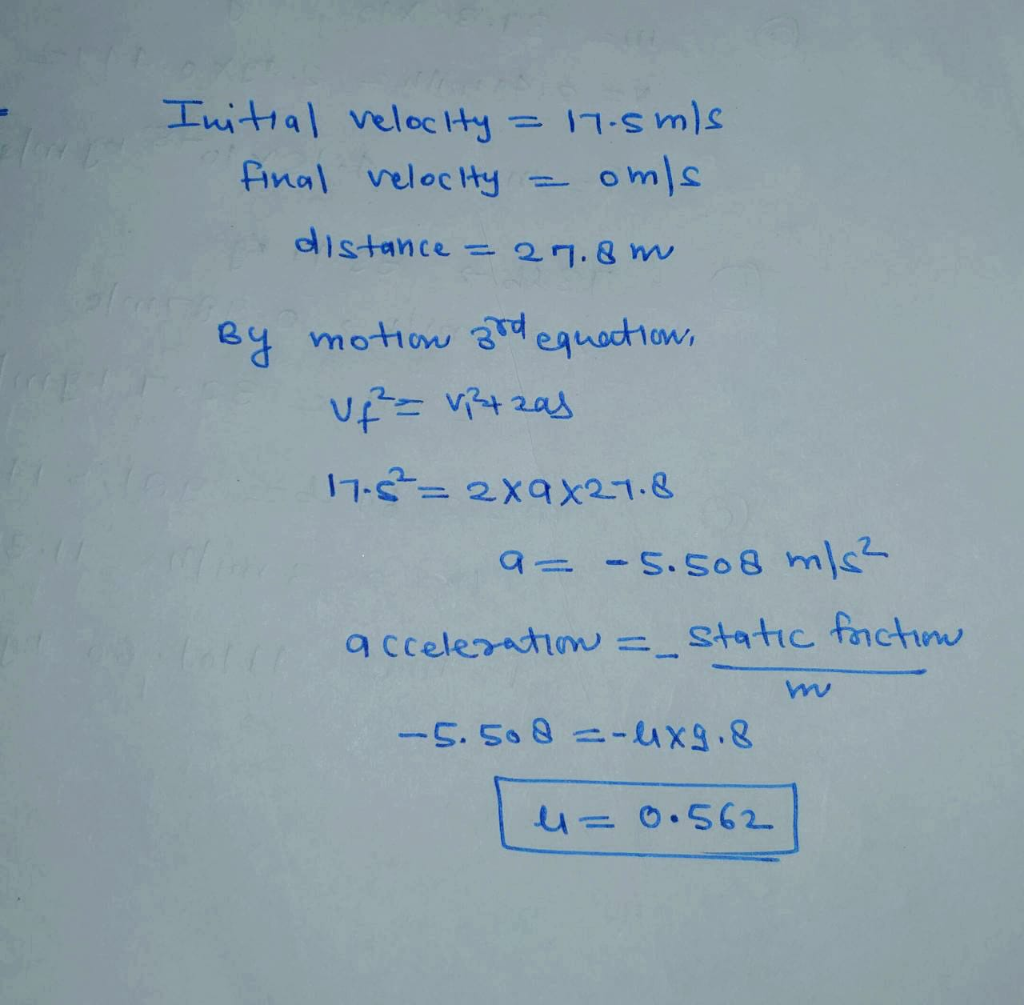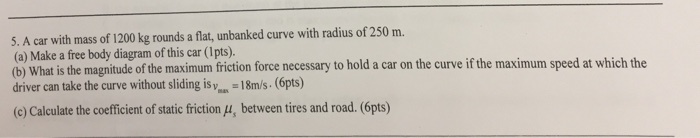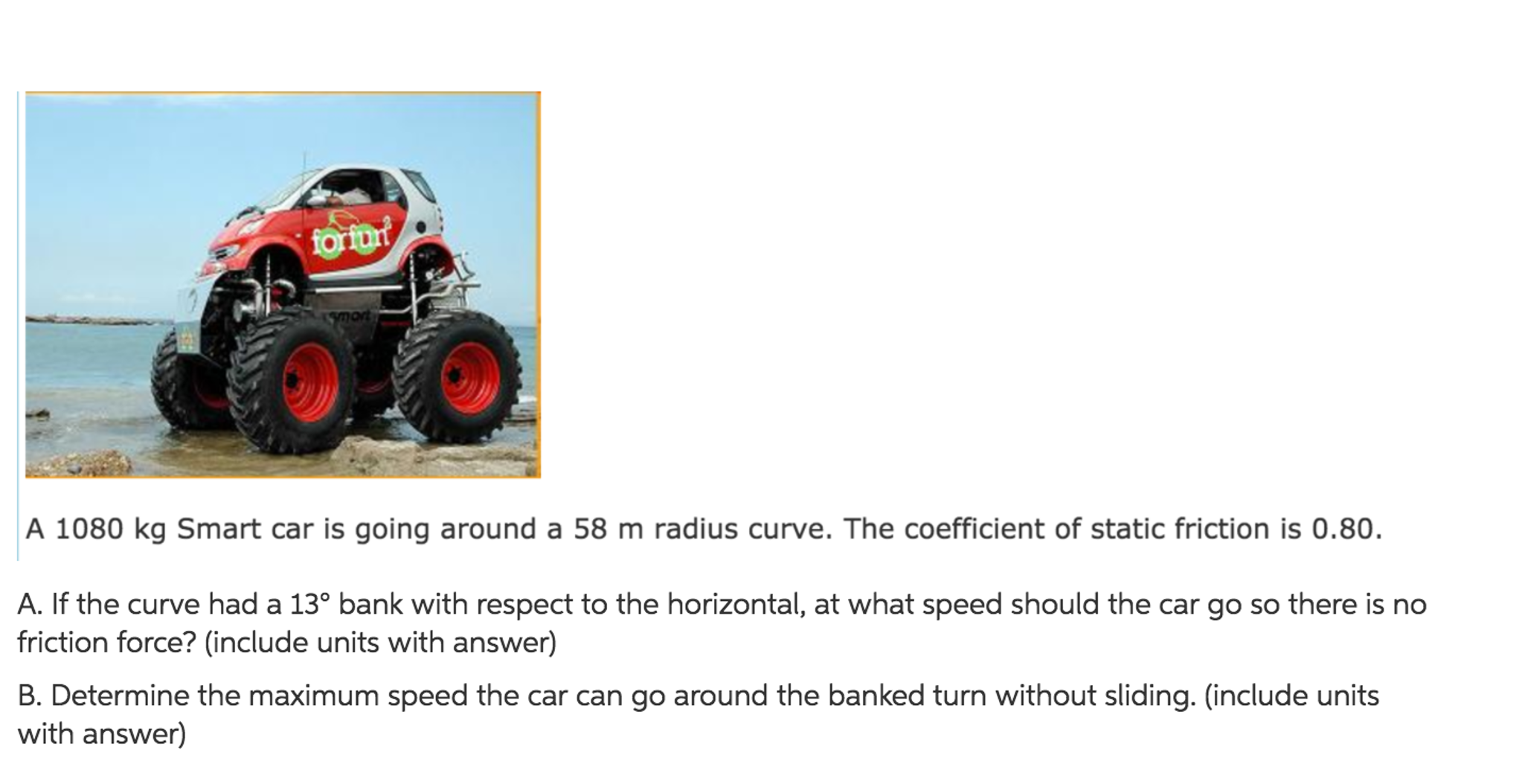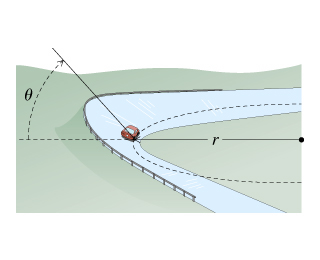Question

# A car with mass 444 kg attempts to make a turn with a radius of 27.8...

A car with mass 444 kg attempts to make a turn with a radius of 27.8 m at a speed of 17.5 m/s on a horizontal surface. What is the minimum value for the static coefficient of friction between the tires and the roadway necessary for the car to make the turn without sliding? Give your answer with 3 significant figures.#### Earn Coins

Coins can be redeemed for fabulous gifts.

Similar Homework Help Questions
• ### A car with mass 444 kg attempts to make a turn with a radius of 27.8...

A car with mass 444 kg attempts to make a turn with a radius of 27.8 m at a speed of 17.5 m/s on a horizontal surface. What is the minimum value for the static coefficient of friction between the tires and the roadway necessary for the car to make the turn without sliding? Give your answer with 3 significant figures.

• ### A car travels at a constant speed of 32.5 mi/h (14.5 m/s) on a level circular...

A car travels at a constant speed of 32.5 mi/h (14.5 m/s) on a level circular turn of radius 49.0 m, as shown in the bird's-eye view in figure a. What minimum coefficient of static friction, μs, between the tires and the roadway will allow the car to make the circular turn without sliding? 1 ) make the circular turn without sliding? 2 ) At what maximum speed can a car negotiate a turn on a wet road with coefficient...

• ### 5. A car with mass of 1200 kg rounds a flat, unbanked curve with radius of...5. A car with mass of 1200 kg rounds a flat, unbanked curve with radius of 250 m. (a) Make a free body diagram of this car (1pts). driver can take the curve without sliding is yos. -18m/s. (6pts) (c) Calculate the coefficient of static friction (u, between tires and road. (6pts) at is the magnitude of the maximum friction force necessary to hold a car on the curve if the maximum speed at which the

• ### Spy Agent 001 is chasing the bad guys. Suddenly, 001 needs to turn his car (m...

Spy Agent 001 is chasing the bad guys. Suddenly, 001 needs to turn his car (m = 1000 kg) around, and completes a turn in the shape of a semi-circle (radius = 60 m) travelling at a constant speed of 27 m/s. a) If the static coefficient of friction between the tires and the pavement is 0.55, find the maximum force of friction before slipping occurs. b) Will the car be able to complete the turn without sliding? c) To...

• ### A 1080 kg Smart car is going around a 58 m radius curve. The coefficient of...A 1080 kg Smart car is going around a 58 m radius curve. The coefficient of static friction is 0.80. If the curve had a 13degree bank with respect to the horizontal, at what speed should the cargo so there is no friction force? (include units with answer) Determine the maximum speed the car can go around the banked turn without sliding, (include units with answer)

• ### The radius of curvature of a highway exit is r = 49.5 m. The surface of...

The radius of curvature of a highway exit is r = 49.5 m. The surface of the exit road is horizontal, not banked What is the minimum required value of the coefficient of static friction between the tires of the car and the surface of the road so that the car can safely exit the highway at a constant speed of 42.0 km/h without sliding?

• ### A car of mass M = 800 kg traveling at 55.0 km/hour enters a banked turn...A car of mass M = 800 kg traveling at 55.0 km/hour enters a banked turn covered with ice. The road is banked at an angle ?, and there is no friction between the road and the car's tires as shown in(Figure 1) . Use g = 9.80 m/s2 throughout this problem. Now, suppose that the curve is level (?=0) and that the ice has melted, so that there is a coefficient of static friction ? between the road and...

• ### A 15 Kg car can make a turn around a curve of radius 20 m on...

A 15 Kg car can make a turn around a curve of radius 20 m on a level (unbanked) road A) draw a free body diagram of forces acting on the car B) what is the force due to friction in terms of weight? C) what is the maximum speed of the car without sliding?

• ### A car of mass M = 1300 kg traveling at 65.0 km/hour enters a banked turn...

A car of mass M = 1300 kg traveling at 65.0 km/hour enters a banked turn covered with ice. The road is banked at an angle θ, and there is no friction between the road and the car's tires as shown in (Figure 1) . Use g = 9.80 m/s2 throughout this problem. r= 91.43 m. Now, suppose that the curve is level (θ=0) and that the ice has melted, so that there is a coefficient of static friction μ...

• ### A 960-kg race car can drive around an unbanked turn at a maximum speed of 45...

A 960-kg race car can drive around an unbanked turn at a maximum speed of 45 m/s without slipping. The turn has a radius of 160 m. Air flowing over the car's wing exerts a downward-pointing force (called the downforce) of 13000 N on the car. (a) What is the coefficient of static friction between the track and the car's tires? (b) What would be the maximum speed if no downforce acted on the car?